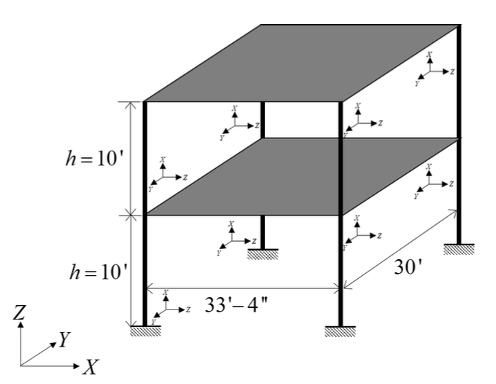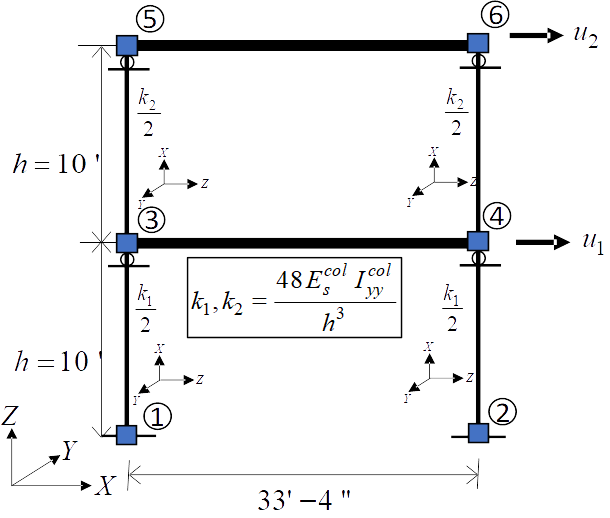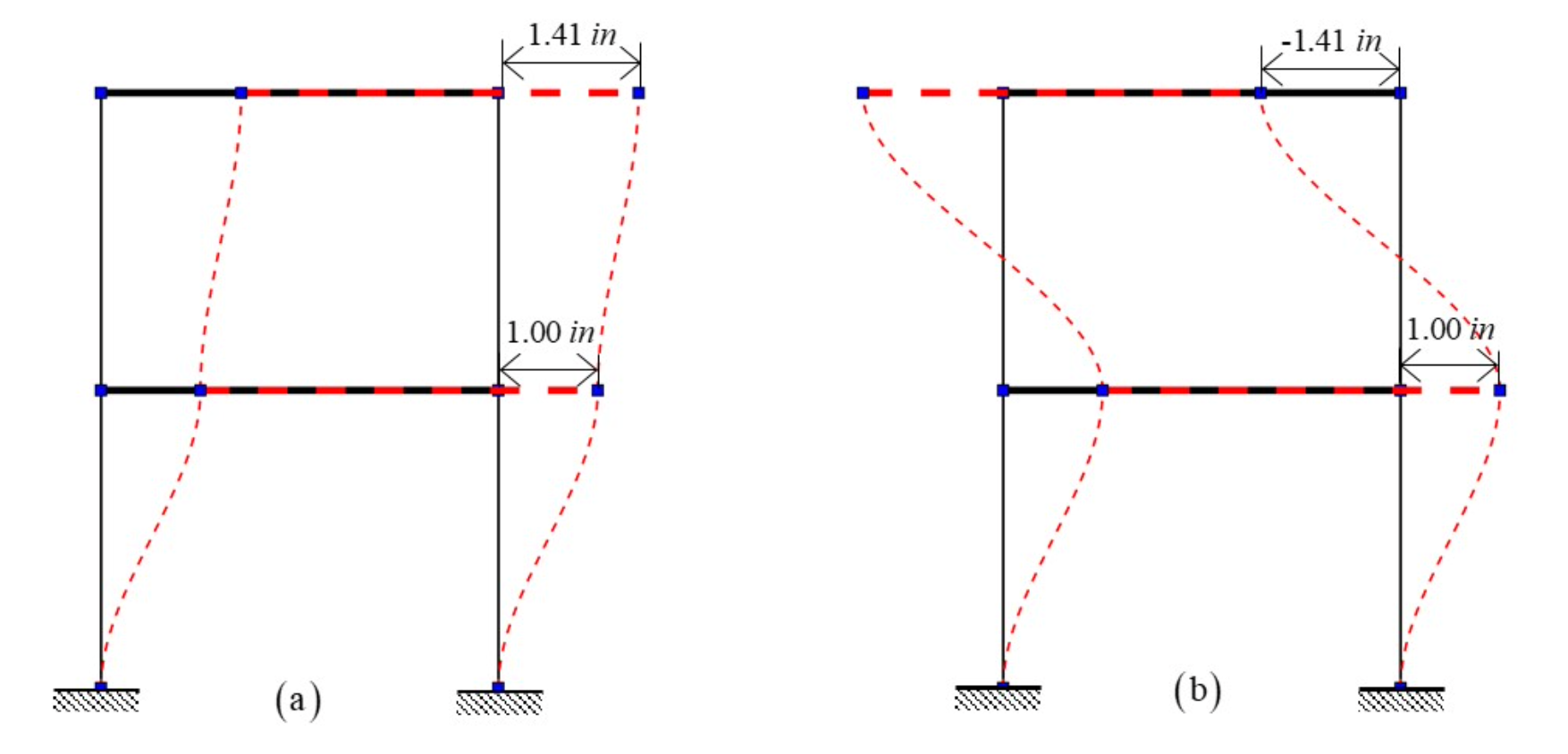# 4.6. Steel Frame: Bayesian Calibration using TMCMC¶

 Problem files qfem-0014/

## 4.6.1. Outline¶

In this example, Bayesian estimation is used to estimate the lateral story stiffnesses of the two stories of a simple steel frame, given data about its mode shapes and frequencies. The transitional Markov chain Monte Carlo algorithm is used to obtain samples from the posterior probability distribution of the lateral story stiffnesses.

## 4.6.2. Problem description¶

This example is provided by Professor Joel Conte and his doctoral students Maitreya Kurumbhati and Mukesh Ramancha from UC San Diego.

### 4.6.2.1. Structural system¶

Consider the two-story building structure shown in Fig. 4.6.2.1.1. Each floor slab is made of a composite metal deck and is supported on the steel columns. These four columns are fixed at the base. The story height is $$h = 10'$$, length of each slab is $$33'4''$$ and $$30'$$ along the X and Y direction, respectively. $$m_1 = 0.52 \ \ kips-s^2/in$$ and $$m_2 = 0.26 \ \ kips-s^2/in$$ are the total mass of floor 1 and floor 2, respectively. For the steel columns, Young’s modulus is $$E_s^{col} = 29000 \ \ ksi$$ and the moment of inertia $$I_{yy}^{col} = 1190 \ \ in^4$$.Fig. 4.6.2.1.1 Steel structural system being studied in this example.

### 4.6.2.2. Finite element model¶

In this example, only the response of the system along the X direction is considered. For modeling purposes, the floor diaphragms are assumed rigid both in plane and in flexure, and the columns are assumed axially rigid. The structure is modeled as a two-story 2D shear building model as shown in Fig. 4.6.2.2.1. The finite element (FE) software framework OpenSees is utilized for modeling and analysis of the considered structural system. The developed FE model consists of 6 nodes and 6 elastic beam-column elements. To simulate the flexural rigidity of the floors, the moment of inertia $$I_{yy}$$ of the horizontal elements is set to a very large number. The horizontal degrees of freedom of node 3 and node 4 are constrained to be equal throughout the analysis to mimic the axial rigidity of floor 1. Similar modeling is performed for floor 2. The vertical displacements of nodes 3, 4, 5, and 6 are constrained to be zero to model the axial rigidity of the columns (see roller supports in Fig. 4.6.2.2.1). After making these modeling assumptions, the only active degrees of freedom of the FE model are the horizontal displacements (translations) of floors 1 and 2, $$u_1$$ and $$u_2$$, respectively, as shown in Fig. 4.6.2.2.1.

A translational mass $$m_1/2$$ and $$m_2/2$$ is lumped at the nodes of floor 1 and 2, respectively, along the X direction. The lateral story stiffnesses $$k_1$$ and $$k_2$$ of story 1 and 2, respectively, are equal to $$48 E_s^{col} I_{yy}^{col}/{h^3}$$. Hence, the story stiffnesses $$k_1$$ and $$k_2$$ are both equal to 958.61 kips/in.Fig. 4.6.2.2.1 Model of the structural system used in finite element analysis.

### 4.6.2.3. Natural vibration frequencies and mode shapes¶

Since the shear building model shown in Fig. 4.6.2.2.1 has only two degrees of freedom, it has two natural modes of vibration. Let $$\lambda_i$$ and $$\phi_i$$ be the $$i^{th}$$ eigenvalue and its corresponding eigenvector, respectively. The two eigenvalues and eigenvectors are obtained by solving the generalized eigenvalue problem of the considered system in OpenSees. The following two eigenvalues are obtained:

(4.6.2.3.1)$\begin{array}{l} \lambda_1 = 1084.06 (rad/s)^2, \quad \lambda_2 = 6318.34 (rad/s)^2 \end{array}$

The corresponding eigenvectors (see degrees of freedom u1 and u2 in Fig. 4.6.2.2.1) are given by:

(4.6.2.3.2)$\begin{split}\phi_1 = \begin{pmatrix}\phi_{11} \\ \phi_{12}\end{pmatrix} = \begin{pmatrix}1.00 \\ 1.41\end{pmatrix} in, \qquad \phi_2 = \begin{pmatrix}\phi_{21} \\ \phi_{22}\end{pmatrix} = \begin{pmatrix}1.00 \\ -1.41\end{pmatrix} in\end{split}$

The eigenvectors in (4.6.2.3.2) are normalized such that the first component is 1.0. The two vibration mode shapes are shown in Fig. 4.6.2.3.1.Fig. 4.6.2.3.1 Natural vibration mode shapes: (a) Mode 1 (b) Mode 2.

### 4.6.2.4. Parameters to be estimated¶

The FE model of a real structural system often consists of parameters that are unknown to some degree. For example, the parameters related to the mass or stiffness or damping of the system might be unknown. The goal of parameter estimation is to estimate such unknown parameters using some measurement data. The measurement data is obtained by using sensors deployed on the real system. To demonstrate the parameter estimation concept/framework on the considered two-story building system, the first and second story story stiffnesses, $$k_{1}$$ and $$k_{2}$$, are assumed to be unknown while the mass parameters are assumed to be known. In this illustration example, the unknown parameter vector $$\mathbf{\theta}=(k_{1}, k_{2})^T$$ is estimated using the first eigenvalue and the first eigenvector data.

### 4.6.2.5. Synthetic data generation¶

In a real-world application, data on the first eigenvalue and the first eigenvector would consist of system identification results obtained from sensor measurement data. Note that the considered two-story building structure (see Fig. 4.6.2.1.1) is used here as a conceptual/pedagogical example and does not exist in the real world. Therefore, sensor measurement data cannot be collected from the system. As a substitute, measurement data (in the form of estimated first eigenvalue and first eigenvector) are artificially simulated for the purpose of this example, i.e., system identification results for $$\lambda_i$$ and $$\phi_i$$ from multiple ambient vibration datasets are simulated. To simulate these system identification results (i.e., measurement data), an eigenvalue analysis of the system is performed assuming the following true principal moment of inertia of the columns:

(4.6.2.5.1)$\mathbf{\theta}^{true} = \left(k_{1}^{true}, k_{2}^{true}\right)^T; \quad k_{1}^{true} = k_{2}^{true} = 958.61 \ \ kips/in.$

The corresponding first eigenvalue and first eigenvector are:

(4.6.2.5.2)$\mathbf{y}^{true} = \left(\lambda_1^{true} = 1084.06 (rad/s)^2, \phi_{12}^{true} = 1.41 in\right)^T$

To simulate system identification results (measurement data), random estimation errors are added to $$\lambda_1^{true}$$ and $$\phi_{12}^{true}$$. The random estimation errors for $$\lambda_1^{true}$$ and $$\phi_{12}^{true}$$ are assumed to be statistically independent, zero-mean Gaussian with 5% coefficient of variation (relative to $$\lambda_1^{true}$$ and $$\phi_{12}^{true}$$). Thus, the standard deviation of the system identification errors for $$\lambda_1^{true}$$ and $$\phi_{12}^{true}$$ are

(4.6.2.5.3)$\sigma_{\lambda_1}^{true} = 0.05*\lambda_1^{true} = 54.203; \quad \sigma_{\phi_{12}}^{true} = 0.05*\phi_{12}^{true} = 0.0705$

Now five independent sets of system identification results (measurement data sets) are simulated as:

(4.6.2.5.4)$\begin{split}\begin{array}{l} \lambda_{1}^{(1)}=1025.21, \quad \lambda_{1}^{(2)}=1138.11, \quad \lambda_{1}^{(3)}=1099.39, \quad \lambda_{1}^{(4)}=1002.41, \quad \lambda_{1}^{(5)}=1052.69 \\ \phi_{12}^{(1)}=1.53, \quad \phi_{12}^{(2)}=1.24, \quad \phi_{12}^{(3)}=1.38, \quad \phi_{12}^{(4)}=1.50, \quad \phi_{12}^{(5)}=1.35 \end{array}\end{split}$

### 4.6.2.6. Parameter estimation setup¶

In this example, it is assumed that the story stiffnesses for the first and second story ($$k_{1}$$ and $$k_{2}$$ respectively) are unknown. The goal is to use the simulated data of eigenvalue and eigenvector measurements to obtain the posterior probability distribution of the story stiffnesses by Bayesian calibration. We will employ the Transitional Markov Chain Monte Carlo (TMCMC) algorithm, to sample from the posterior joint probability distribution of $$k_{1}$$ and $$k_{2}$$.

We define the following prior probability distributions for the unknown quantities of interest:

1. First story stiffness, k1: Uniform distribution with a lower bound $$(L_B)$$ of $$766.89 \ \mathrm{kips/in}$$, and an upper bound $$(U_B)$$ of $$2108.94 \ \mathrm{kips/in}$$,

2. Second story stiffness, k2: Uniform distribution with a lower bound $$(L_B)$$ of $$383.44 \ \mathrm{kips/in}$$, and an upper bound $$(U_B)$$ of $$1150.33 \ \mathrm{kips/in}$$.

A Gaussian (a.k.a. Normal) likelihood model is employed by default in quoFEM. This is done by assuming that the errors (i.e. the differences between the finite element prediction of the modal properties and the simulated measurement data) follow a zero-mean Gaussian distribution. The components of the error vector are assumed to be statistically independent. The errors in the prediction of a particular response quantity are assumed to be identically distributed across experiments. Under these assumptions, the standard deviations of the error for each response quantity are the only unknown parameters of the Gaussian distribution employed in the likelihood model, and their values are also estimated from the data. Therefore, in this problem, there are two additional parameters $$\sigma_{\lambda_{1}}$$ and $$\sigma_{\phi_{12}}$$, which are also estimated. The prior probability distributions for these additional parameters are automatically set by quoFEM.

### 4.6.2.7. Files required¶

The exercise requires two script files. The user should download these files and place them in a new folder.

Warning

Do not place the files in your root, downloads, or desktop folder as when the application runs it will copy the contents on the directories and subdirectories containing these files multiple times. If you are like us, your root, Downloads or Documents folders contains a lot of files.

1. model.tcl - This is an OpenSees script written in tcl which builds the finite element model and conducts the analysis. This script creates a file called results.out when it runs. This file contains the first eigenvalue and the second component of the first eigenvector obtained from finite element analysis of the structure.

2. eigData.csv - This is the calibration data file, which contains the synthetically generated data in five rows and two columns, the contents of which are shown below.

1025.21, 1.53
1138.11, 1.24
1099.39, 1.38
1002.41, 1.50
1052.69, 1.35


Note

Since the tcl script creates a results.out file when it runs, no postprocessing script is needed.

## 4.6.3. UQ workflow¶

Note

Selecting the Steel Frame: Bayesian Calibration using TMCMC example in the quoFEM Examples menu will autopopulate all the input fields required to run this example. The procedure outlined below demonstrates how to manually set up this problem in quoFEM.

The steps involved are as follows:

1. Start the application and the UQ panel will be highlighted. In the UQ Engine drop down menu, select the UCSD_UQ engine. In the Method category drop down menu the Transitional Markov chain Monte Carlo option will be highlighted. Enter the values in this panel as shown in the figure below. If manually setting up this problem, choose the path to the file containing the calibration data on your system.

1. Next select the FEM panel from the input panel selection. This will default to the OpenSees FEM engine. In the Input Script field, enter the path to the model.tcl file or select Choose and navigate to the file.

1. Next select the RV tab from the input panel. This panel should be pre-populated with two random variables named k1 and k2. If not, press the Add button twice to create two fields to define the input random variables. Enter the same variable names (k1 and k2), as required in the model.tcl script.

For each variable, specify the prior probability distribution - from the Distribution drop down menu, select Uniform and then provide the lower bounds and upper bounds shown in the figure below.

1. In the QoI panel, enter 2 variable names for the two quantities output from the model.

Note

For this particular problem setup in which the user is not using a postprocessing script, the user may specify any names for the QoI variables. They are only being used by Dakota to return information on the results.

5. Next click on the Run button. This will cause the backend application to launch the UCSD_UQ engine, which performs Bayesian calibration using the TMCMC algorithm. When done, the RES tab will be selected and the results will be displayed as shown in the figure below. The results show the first four moments of the posterior marginal probability distribution of $$k_1$$ and $$k_2$$. The true value of both $$k_1$$ and $$k_2$$ is 958.61 kips/in. Also shown are the moments of the estimated aditional error parameters per response quantity and finally, the moments of the outputs corresponding to the samples from the posterior probability distribution of the parameters.

If the user selects the Data Values tab in the results panel, they will be presented with both a graphical plot and a tabular listing of the data.

Various views of the graphical display can be obtained by left and right clicking in the columns of the tabular data.

If a singular column of the tabular data is pressed with both right and left buttons a histogram and CDF will be displayed, as shown in the figures below.